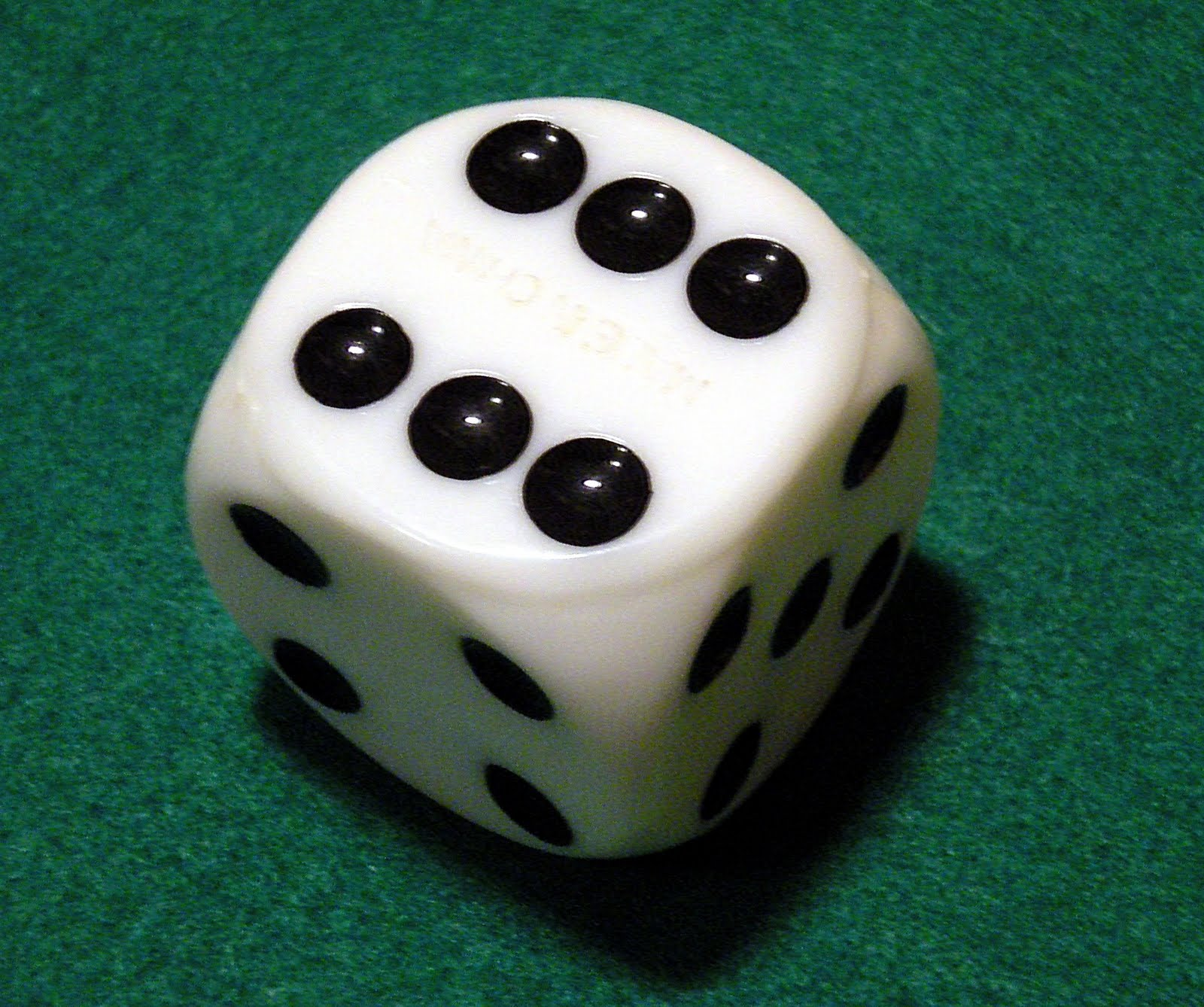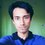Imagine that you have a loaded die (that’s the singular of ‘dice’). This means the die is biased. It’s not fair. If you roll it, the probability that you’ll get a $4$ is higher than the probability of getting any other number. You roll the die a few times and analyze the data.

Decide which of the following is more likely to happen:

$A. \quad 2, 5, 3, 4, 6$ $B. \quad 4, 2, 5, 3, 4, 6$

Drop a comment below with your answer, and please do not explain your answer because I don’t want anyone to get influenced by other peoples’ comments. Just a simple $A$ or a $B$ will suffice.

I’m going to bed now and when I wake up the next day I hope to see a lot of comments! :)

Until then!Note by Mursalin Habib
6 years, 10 months ago

This discussion board is a place to discuss our Daily Challenges and the math and science related to those challenges. Explanations are more than just a solution — they should explain the steps and thinking strategies that you used to obtain the solution. Comments should further the discussion of math and science.

When posting on Brilliant:

• Use the emojis to react to an explanation, whether you're congratulating a job well done , or just really confused .
• Ask specific questions about the challenge or the steps in somebody's explanation. Well-posed questions can add a lot to the discussion, but posting "I don't understand!" doesn't help anyone.
• Try to contribute something new to the discussion, whether it is an extension, generalization or other idea related to the challenge.

MarkdownAppears as
*italics* or _italics_ italics
**bold** or __bold__ bold
- bulleted- list
• bulleted
• list
1. numbered2. list
1. numbered
2. list
Note: you must add a full line of space before and after lists for them to show up correctly
paragraph 1paragraph 2

paragraph 1

paragraph 2

[example link](https://brilliant.org)example link
> This is a quote
This is a quote
    # I indented these lines
# 4 spaces, and now they show
# up as a code block.

print "hello world"
# I indented these lines
# 4 spaces, and now they show
# up as a code block.

print "hello world"
MathAppears as
Remember to wrap math in $$ ... $$ or $ ... $ to ensure proper formatting.
2 \times 3 $2 \times 3$
2^{34} $2^{34}$
a_{i-1} $a_{i-1}$
\frac{2}{3} $\frac{2}{3}$
\sqrt{2} $\sqrt{2}$
\sum_{i=1}^3 $\sum_{i=1}^3$
\sin \theta $\sin \theta$
\boxed{123} $\boxed{123}$

Sort by:

Option 1: A

Upvote this comment if you think it is right.

- 6 years, 10 months ago

Option 2: B

Upvote this comment if you think it is right.

- 6 years, 10 months ago

Option B

- 6 years, 10 months ago

I say A.

- 6 years, 9 months ago

B

- 6 years, 10 months ago

I see it's possible that both are equally likely to happen. But one option cannot be more likely than the other; it's either equally likely or less likely depending on the probability distribution.

- 6 years, 10 months ago

But one option cannot be more likely than the other;

Why is that? If something is less likely to happen then something else, then that something else is more likely to happen than the first something.

- 6 years, 9 months ago

I mean one particular option cannot be more likely than the other one, not any one option cannot be more likely. Ambiguity; my bad.

- 6 years, 9 months ago

B

- 6 years, 10 months ago

Option A

- 6 years, 10 months ago

@Mursalin Habib It has been a day!

- 6 years, 9 months ago

A

- 6 years, 9 months ago

Because apparently this one person's "day" meaning 15 days in real world, I decide to screw it and give my reasoning. Here, $P(A), P(B), P(4)$ are probabilities of getting the sequence $A$, the sequence $B$, and the throw $4$ in that order.

$A$ is more likely. Note that $P(B) = P(4) \cdot P(A) \le 1 \cdot P(A) = P(A)$, so the probability of getting $B$ is less than or equal to $A$.

- 6 years, 9 months ago